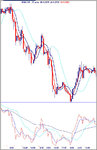#### masao

##### Junior member
42 0
I realize that this is nothing new for most using TradeStation but I noticed that there are some using the RSI in the forex chat that may or may not have this code for an RSI wAvgs

plot1(rsi(close,14),"plot1");
plot2(xaverage((rsi(close,14)),5),"plot2");
plot3[-5](xaverage((rsi(close,14)),13),"plot3");
plot4(xaverage((rsi(close,14)),55),"plot4");

Regards,

#### Attachments

•RSI wAvgs.jpg
102.7 KB · Views: 237